# Math

You are currently browsing the archive for the Math category.

## Gauss’s continued fraction

Gauss did a lot of math, but sometimes I am surprised when I find something new to me done by Gauss

$\tan(x) = { 1 \over{1/x \ – {1\over{3/x \ – {1\over{5/x\ – \ldots}}}}}}$

## Topological Sorting

Given a directed a-cyclic graph (DAG) find an ordering of the nodes which is consistent with the directed edges.  This is called Topological Sorting and several algorithms exist.

## Why are Gaussian Distributions Great?

Gaussian distributions are the most “natural” distributions. They show up everywhere. Here is a list of the properties that make me think that Gaussians are the most natural distributions:

• The sum of several random variables (like dice) tends to be Gaussian. (Central Limit Theorem).
• There are two natural ideas that appear in Statistics, the standard deviation and the maximum entropy principle. If you ask the question, “Among all distributions with standard deviation 1 and mean 0, what is the distribution with maximum entropy?” The answer is the Gaussian.
• Randomly select a point inside a high dimensional hypersphere. The distribution of any particular coordinate is approximately Gaussian. The same is true for a random point on the surface of the hypersphere.
• Take several samples from a Gaussian Distribution. Compute the Discrete Fourier Transform of the samples. The results have a Gaussian Distribution. I am pretty sure that the Gaussian is the only distribution with this property.
• The eigenfunctions of the Fourier Transforms are products of polynomials and Gaussians.
• The solution to the differential equation y’ = -x y is a Gaussian. This fact makes computations with Gaussians easier. (Higher derivatives involve Hermite polynomials.)
• I think Gaussians are the only distributions closed under multiplication, convolution, and linear transformations.
• Maximum likelihood estimators to problems involving Gaussians tend to also be the least squares solutions.
• I think all solutions to stochastic differential equations involve Gaussians. (This is mainly a consequence of the Central Limit Theorem.
• “The normal distribution is the only absolutely continuous distribution all of whose cumulants beyond the first two (i.e. other than the mean and variance) are zero.” – Wikipedia.
• For even n, the nth moment of the Gaussian is simply an integer multiplied by the standard deviation to the nth power.
• Many of the other standard distributions are strongly related to the Gaussian (i.e. binomial, Poisson, chi-squared, Student t, Rayleigh, Logistic, Log-Normal, Hypergeometric …)
• “If X1 and X2 are independent and their sum X1 + X2 is distributed normally, then both X1 and X2 must also be normal.” — From the Wikipedia.
• “The conjugate prior of the mean of a normal distribution is another normal distribution.” — From the Wikipedia.
• When using Gaussians, the math is easier.
• The Erdős–Kac theorem implies that the distribution of the prime factors of a “random” integer is Gaussian.
• The velocities of random molecules in a gas are distributed as a Gaussian. (With standard deviation = $z*\sqrt{ k\, T / m}$ where $z$ is a “nice” constant, $m$ is the mass of the particle, and $k$ is Boltzmann’s constant.)
• “A Gaussian function is the wave function of the ground state of the quantum harmonic oscillator.” — From Wikipedia
• Kalman Filters.
• The Gauss–Markov theorem.

## “Old and New Matrix Algebra Useful for Statistics”

Sometimes I can’t remember the formulas for matrix calculus.  Fortunately there are some good references on the web.  One of my favorites is “Old and New Matrix Algebra Useful for Statistics” (Minka 2000 1, 2).

## Proofs without Words

On mathoverflow, they have this wonderful question “Can you give examples of proofs without words?”.  The answers are beautiful. They visually “prove”

$$1 + 2 + 3 + \cdots + n = n (n + 1) / 2 = \left({ \begin{matrix} n \\ 2 \end{matrix}}\right),$$

$$32.5 = 31.5,$$

$$1^2 + 2^2 + 3^2 + \cdots + n^2 = n(n + 1)(n + 1/2)/3,$$

$$F^2_0 + F^2_1 + \cdots + F^2_n = F_n F_{n+1},$$

with $F_0 = 1$ for Fibonacci numbers $F_n$, and more than 15 other facts visually.

## Rolling Dice Mod 3

It is fairly obvious if you think about it and if you are a mathematician, but I was surprised by this theorem.

Theorem:  Roll any number of dice all of which can have any (finite) number of sides except at least one die which is the standard six sided die.  The probability that the sum is divisible by three is exactly 1/3.  Also, the probability that the sum is odd is exactly 1/2.

Corollary:  Roll any number of six sided dice. The probability that the sum is divisible by 3 is 1/3.  The probability that the sum is even is exactly 1/2.

## Venn Diagrams

Carl sent me a link to a Venn Diagrams post, so that got me thinking. A Venn Diagram with $n$ atoms has to represent $2^n$ regions. For example if $n$ is $2$, then you have the standard Venn Diagram below.Each time you increase $n$ by one, you double the number of regions.  This makes me think of binary codes and orthogonal functions. Everybody’s favorite orthogonal functions are the trig functions, so you should be able to draw Venn diagrams with wavy trig functions. Here was my first try.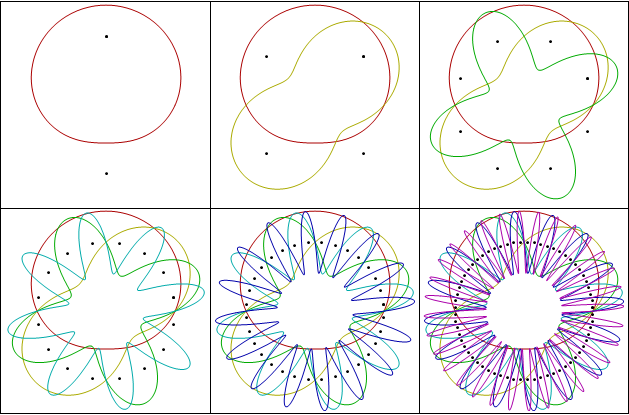Those seemed kind of busy, so I dampened the amplitude on the high frequencies (making the slopes that same and possibly increasing artistic appeal.)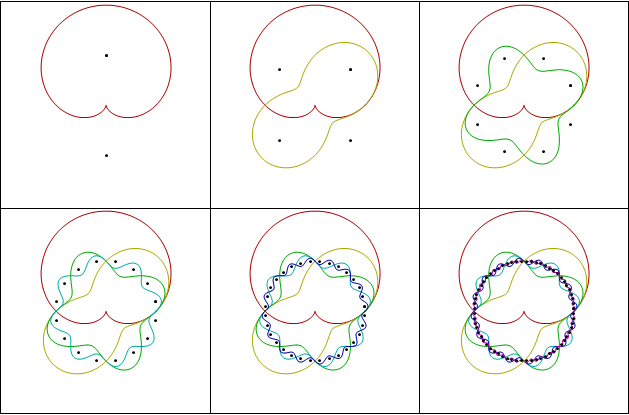I really like the last one.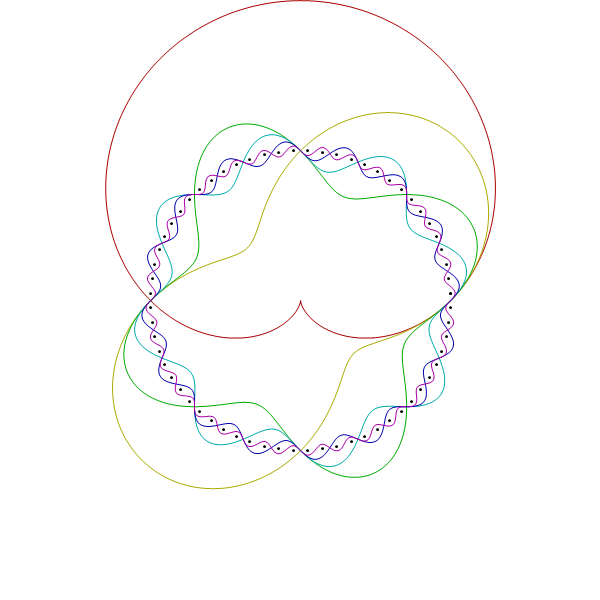## Andrew’s Commentary on Even Denominator Fractions

My Friend Andrew wrote:

Initially, I thought it was dependent on your definition of “random rational number”, but then I saw your second example that removed duplicates (1/2, 2/4, etc..).

I tried a different method by sampling from a real distribution and then rationalizing it:

Mean@Mod[Denominator@Rationalize[#, 1*^-6] & /@ RandomReal[{0, 1}, 100000], 2]


Then, I tried changing the distribution, and still get the same result:

Mean@Mod[Denominator@Rationalize[#, 1*^-6] & /@ RandomReal[NormalDistribution[0, 1], 100000], 2]


— Andrew

## 1/3 of all fractions have an even denominator!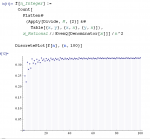What percentage of “random fractions” have an even denominator? My friend Pig told me that the answer is around 1/3. (At least the answer is 1/3 for two somewhat natural definitions of the term “random fraction”.) Here is the experimental “proof” in Mathematica.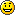Mathematica input for test #1:

percentage[v_List, pred_] := N[ Length[Select[v, pred]] / Length[v] ];

percentage[ Table[ RandomInteger[{1, 1000}] / RandomInteger[{1, 1000}],
{100000} ], EvenQ[Denominator[#]] ]


Output for test #1:

0.33343

Mathematica input for test #2:

percentage[ Table[i/j, {i, 1000}, {j, 1000}] // Flatten // Union,
EvenQ[Denominator[#]] ]


Output for test #2 :

0.333443